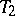# Darbo fixed-point theorem

The notion of "measure of non-compactness" was first introduced by C. Kuratowski [a1]. For any bounded set $B$ in a metric space its measure of non-compactness, denoted by $\alpha ( B )$, is defined to be the infimum of the positive numbers $d$ such that $B$ can be covered by a finite number of sets of diameter less than or equal to $d$.

Another measure of non-compactness is the ball measure $\mu ( B )$, or Hausdorff measure, which is defined as the infimum of the positive numbers $r$ such that $B$ can be covered by a finite number of balls of radii smaller than $r$. See also Hausdorff measure.

Roughly speaking, a measure of non-compactness is some function defined on the family of all non-empty bounded subsets of a given metric space such that it is equal to zero on the whole family of relatively compact sets.

G. Darbo used a measure of non-compactness to investigate operators whose properties can be characterized as being intermediate between those of contraction and compact mappings (cf. also Compact mapping; Compact operator; Contraction). He was the first to use the index $\alpha$ in the theory of fixed points [a2]. Darbo's fixed-point theorem is a generalization of the well-known Schauder fixed-point theorem (cf. also Schauder theorem). It states that if $S$ is a non-empty bounded closed convex subset of a Banach space $X$ and $T : S \rightarrow S$ is a continuous mapping such that for any set $E \subset S$,

\begin{equation} \tag{a1} \alpha ( T E ) \leq k \alpha ( E ), \end{equation}

where $k$ is a constant, $0 \leq k < 1$, then $T$ has a fixed point. This theorem is true for the measure $\mu$ also.

Note that every completely-continuous mapping (or compact mapping; cf. also Completely-continuous operator) satisfies (a1) with $k = 0$, while all Lipschitz mappings with constant $k$ (cf. Lipschitz condition) also satisfy (a1). Further, mappings that are not completely continuous but satisfy the condition (a1) are of the form $T = T _ { 1 } + T _ { 2 }$, where $T _ { 1 }$ is completely continuous andsatisfies the Lipschitz condition with constant $k$. The significance of this type of mapping is due to the fact that compactness of either the domain or the range is not required.

Methods for determining the value of $\mu ( B )$ for a given set $B$ in a Banach space are given in [a3].

Darbo's fixed-point theorem is useful in establishing the existence of solutions of various classes of differential equations, especially for implicit differential equations, integral equations and integro-differential equations, see [a3]. It is also used to study the controllability problem for dynamical systems represented by implicit differential equations [a4].

How to Cite This Entry:
Darbo fixed-point theorem. Encyclopedia of Mathematics. URL: http://encyclopediaofmath.org/index.php?title=Darbo_fixed-point_theorem&oldid=50713
This article was adapted from an original article by Krishnan Balachandran (originator), which appeared in Encyclopedia of Mathematics - ISBN 1402006098. See original article